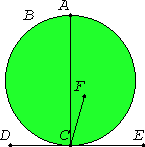# Proposition 19

If a straight line touches a circle, and from the point of contact a straight line is drawn at right angles to the tangent, the center of the circle will be on the straight line so drawn.
I.11

For let a straight line DE touch the circle ABC at the point C. Draw CA from C at right angles to DE.I say that the center of the circle is on AC.

For suppose it is not, but, if possible, let F be the center, and join CF.

III.18

Since a straight line DE touches the circle ABC, and FC has been joined from the center to the point of contact, FC is perpendicular to DE. Therefore the angle FCE is right.

But the angle ACE is also right, therefore the angle FCE equals the angle ACE, the less equals the greater, which is impossible.

Therefore F is not the center of the circle ABC.

Similarly we can prove that neither is any other point except a point on AC.

Therefore if a straight line touches a circle, and from the point of contact a straight line is drawn at right angles to the tangent, the center of the circle will be on the straight line so drawn.

Q.E.D.

## Guide

This proposition is used in proposition III.32.